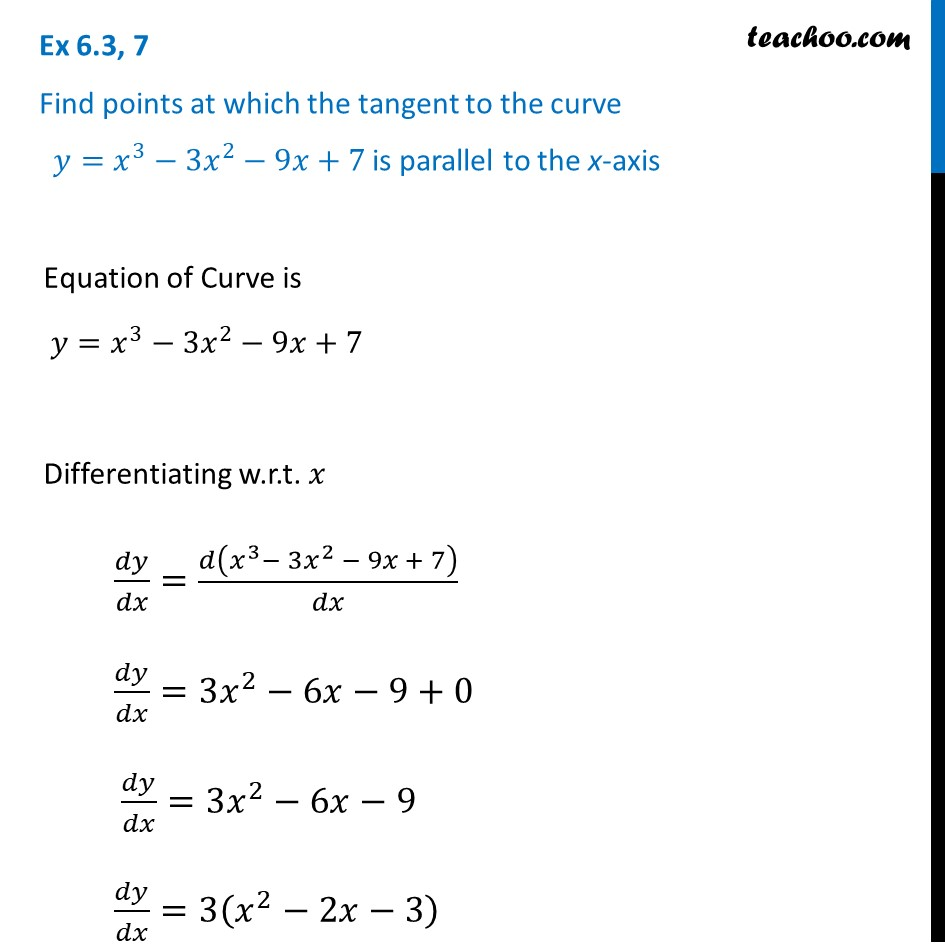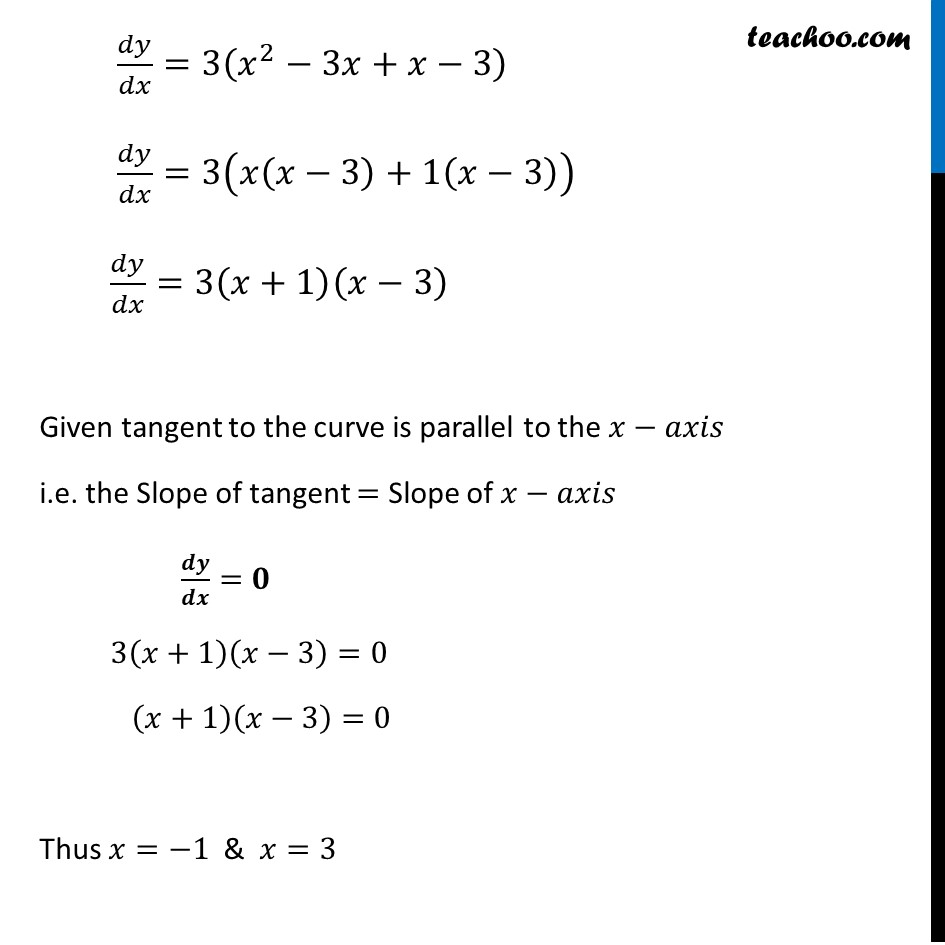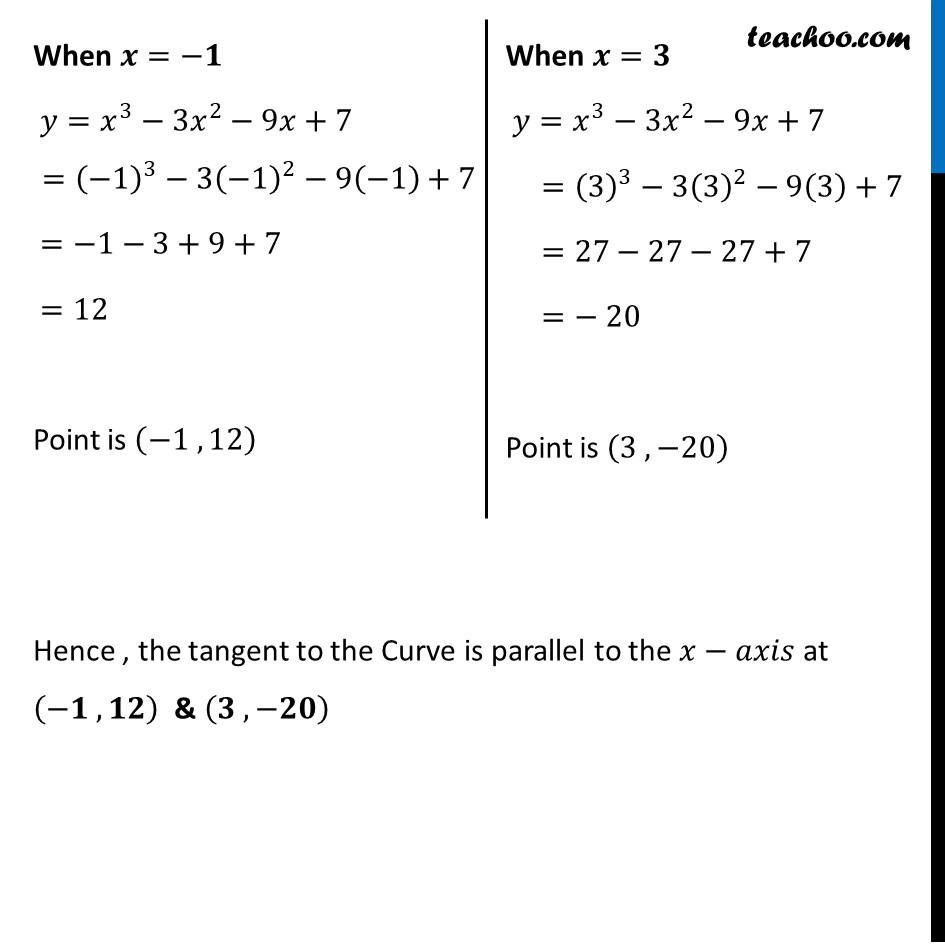Finding point when tangent is parallel/ perpendicular

Chapter 6 Class 12 Application of Derivatives
Concept wiseIntroducing your new favourite teacher - Teachoo Black, at only ₹83 per month

### Transcript

Ex 6.3, 7 Find points at which the tangent to the curve 𝑦=𝑥^3−3𝑥^2−9𝑥+7 is parallel to the x-axisEquation of Curve is 𝑦=𝑥^3−3𝑥^2−9𝑥+7 Differentiating w.r.t. 𝑥 𝑑𝑦/𝑑𝑥=𝑑(𝑥^3− 3𝑥^2 − 9𝑥 + 7)/𝑑𝑥 𝑑𝑦/𝑑𝑥=3𝑥^2−6𝑥−9+0 𝑑𝑦/𝑑𝑥=3𝑥^2−6𝑥−9 𝑑𝑦/𝑑𝑥=3(𝑥^2−2𝑥−3) 𝑑𝑦/𝑑𝑥=3(𝑥^2−3𝑥+𝑥−3) 𝑑𝑦/𝑑𝑥=3(𝑥(𝑥−3)+1(𝑥−3)) 𝑑𝑦/𝑑𝑥=3(𝑥+1)(𝑥−3) Given tangent to the curve is parallel to the 𝑥−𝑎𝑥𝑖𝑠 i.e. the Slope of tangent = Slope of 𝑥−𝑎𝑥𝑖𝑠 𝒅𝒚/𝒅𝒙=𝟎 3(𝑥+1)(𝑥−3)=0 (𝑥+1)(𝑥−3)=0 Thus 𝑥=−1 & 𝑥=3 When 𝒙=−𝟏 𝑦=𝑥^3−3𝑥^2−9𝑥+7 =(−1)^3−3(−1)^2−9(−1)+7 =−1−3+9+7 =12 Point is (−1 , 12) When 𝒙=𝟑 𝑦=𝑥^3−3𝑥^2−9𝑥+7 =(3)^3−3(3)^2−9(3)+7 =27−27−27+7 =− 20 Point is (3 , −20) Hence , the tangent to the Curve is parallel to the 𝑥−𝑎𝑥𝑖𝑠 at (−𝟏 , 𝟏𝟐) & (𝟑 , −𝟐𝟎)